Next: Parallactic inequality Up: Lunar motion Previous: Variation

# Annual equation

Next, let us consider terms in the solution of the lunar equations of motions that depend linearly on the solar eccentricity,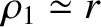.

According to Equations (11.135) and (11.147),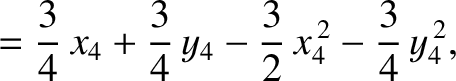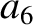(11.231) and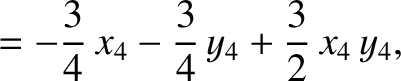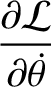(11.232)

It follows from Equations (11.192), (11.193), and (11.201) that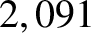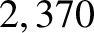(11.233) and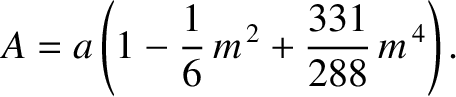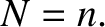(11.234)

According to Equations (11.136) and (11.148),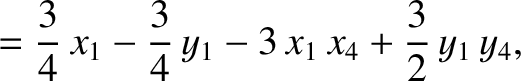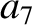(11.235) and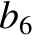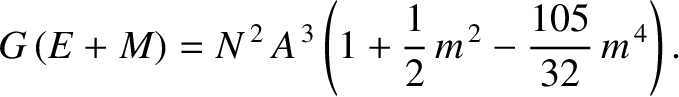(11.236)

It follows from Equations (11.192), (11.193), and (11.202) that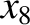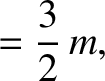(11.237) and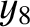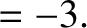(11.238)

Finally, according to Equations (11.137) and (11.149),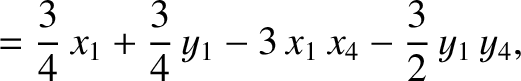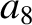(11.239) and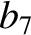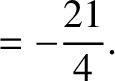(11.240)

Equations (11.192), (11.193), and (11.203) yield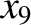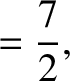(11.241) and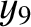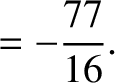(11.242)

It follows from Equations (11.122)-(11.124), (11.167)-(11.169), and (11.179)-(11.181), as well as the previous expressions for,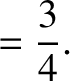,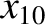,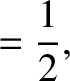,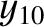, and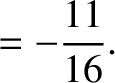, that the net perturbation of the lunar orbit due to terms in the solution of the lunar equations of motion that depend linearly onis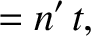(11.243)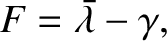(11.244) and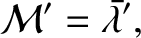(11.245)

The previous expression are accurate to.

The first term on the right-hand side of Equation (11.244) is known as the annual equation, and is caused by a combination of the perturbing action of the Sun and the slight eccentricity (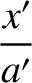) of the apparent solar orbit about the Earth-Moon barycenter. The annual equation attains its maximum amplitude when the Earth (or, rather, the Earth-Moon barycenter) is halfway between its perihelion and its aphelion points (i.e., whenor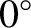). Conversely, the amplitude of the annual equation is zero when the Earth passes through its perihelion or its aphelion points (i.e., whenor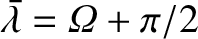). According to Equation (11.244), the annual equation generates a perturbation in the lunar ecliptic longitude that oscillates with a period of a solar year, and has an amplitude (calculated usingand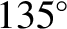) of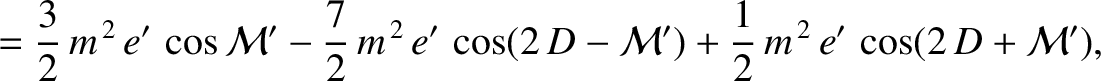arc seconds. As before, the oscillation period is in good agreement with observations, whereas the amplitude is somewhat inaccurate [it should be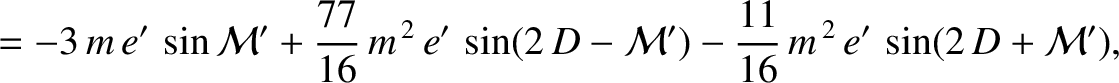arc seconds (Chapront-Touzé and Chapront 1988)] because of the omission of higher-order (inand) contributions.Next: Parallactic inequality Up: Lunar motion Previous: Variation
Richard Fitzpatrick 2016-03-31